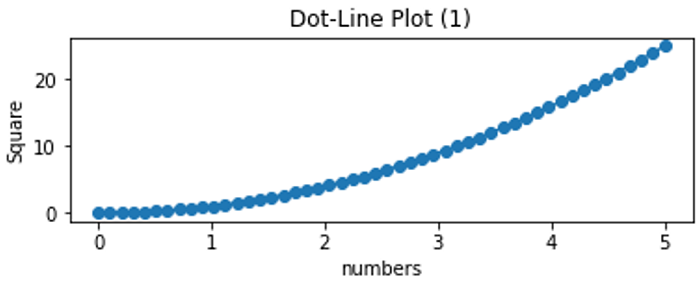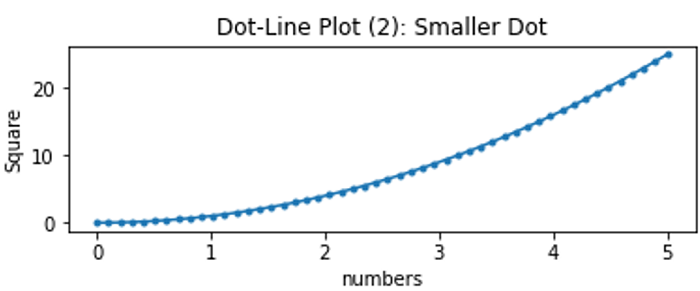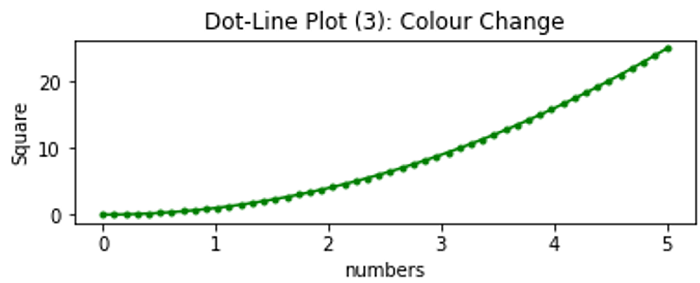# Python | Dot-Line Plotting

Python | Dot-Line Plotting: In this tutorial, we are going to learn about the dot-line plotting and its Python implementation.
Submitted by Anuj Singh, on July 11, 2020

A mixture of dot and line plot is called a Dot-Line plot. Each dot is connected through a line and it is the next version of the line plot. It maintains the discrete property of the points and also represents the correlation between consecutive points. It makes data visualization much better than an individual line or dot plot. Matplotlib provides this feature and with the following examples, we can better understand the implementation.

## 1) Standard Dot-Line Plot

Syntax:

```plt.plot(x, y,'o-')
```
• x - names/numeric distribution
• y - length of the bar
• o- - instruction for dot-line## 2) Dot-Line Plot with Smaller Dot

Syntax:

```plt.plot(x, y,'o-')
```
• x - names/numeric distribution
• y - length of the bar
• .- - instruction for dot-line with small dot## 3) Dot-Line Plot with Different Color

Syntax:

```plt.plot(x, y,'o-')
```
• x - names/numeric distribution
• y - length of the bar
• g.- - instruction for dot-line with small dot green color## Python code for dot-line plotting

```import numpy as np
import matplotlib.pyplot as plt

x = np.linspace(0.0, 5.0)

y = x*x

# default Plot
plt.subplot(2, 1, 1)
plt.plot(x, y, 'o-')
plt.title('Dot-Line Plot (1)')
plt.ylabel('Square')
plt.xlabel('numbers')
plt.show()

# Smaller dot
plt.subplot(2, 1, 2)
plt.plot(x, y, '.-')
plt.title('Dot-Line Plot (2): Smaller Dot')
plt.xlabel('numbers')
plt.ylabel('Square')
plt.show()

#colour Change
plt.subplot(2, 1, 2)
plt.plot(x, y, 'g.-')
plt.title('Dot-Line Plot (3): Colour Change')
plt.xlabel('numbers')
plt.ylabel('Square')
plt.show()
```

Output:

```Output is as figure
```

Languages: » C » C++ » C++ STL » Java » Data Structure » C#.Net » Android » Kotlin » SQL
Web Technologies: » PHP » Python » JavaScript » CSS » Ajax » Node.js » Web programming/HTML
Solved programs: » C » C++ » DS » Java » C#
Aptitude que. & ans.: » C » C++ » Java » DBMS
Interview que. & ans.: » C » Embedded C » Java » SEO » HR
CS Subjects: » CS Basics » O.S. » Networks » DBMS » Embedded Systems » Cloud Computing
» Machine learning » CS Organizations » Linux » DOS
More: » Articles » Puzzles » News/Updates